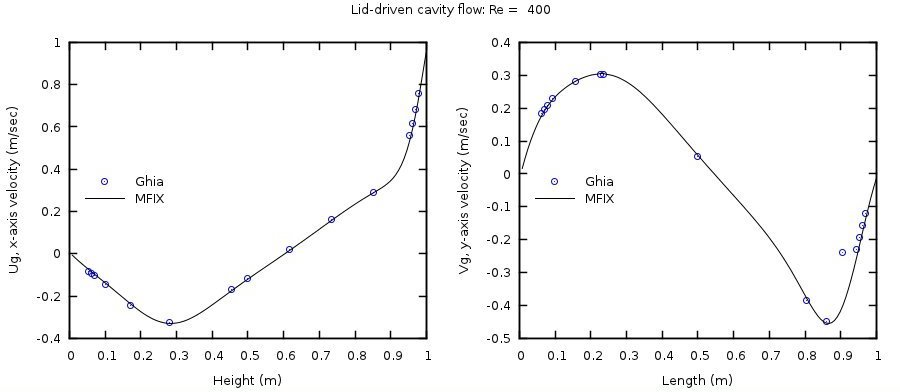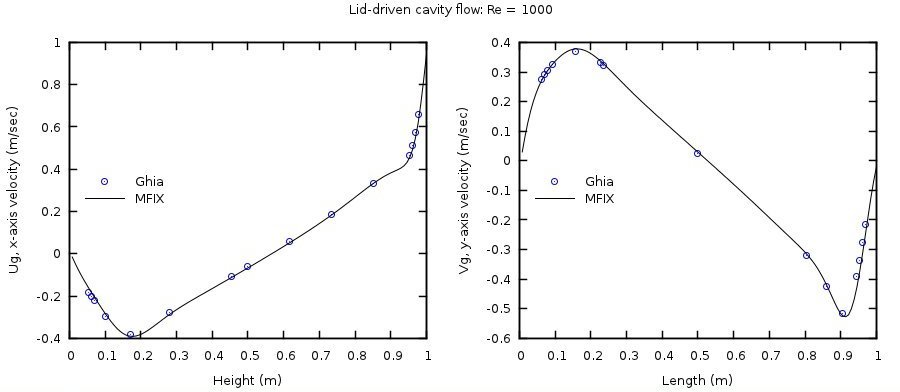# 3.3. FLD03: Steady, lid-driven square cavity¶

## 3.3.1. Description¶

Lid-driven flow in a 2D square cavity in the absence of gravity is illustrated in Fig. 3.6. The problem definition follows the work of Ghia et al.  where the domain is bounded on three sides with stationary walls while one wall, the lid, is prescribed a constant velocity. The cavity is completely filled with a fluid of selected viscosity and the flow is assumed to be incompressible and laminar.Fig. 3.6 Schematic of the lid-driven square cavity.

## 3.3.2. Setup¶

########################################################################
#                                                                      #
# Author: M. Syamlal                                    Date: Dec 1999 #
# Revised: A. Choudhary and J. Musser                   Date: Jun 2015 #
#                                                                      #
# A top wall driven cavity with only fluid phase present in the        #
# absence of gravitational forces.                                     #
#                                                                      #
# REF: Ghia U., Ghia K.N., and Shin C.T., High-Re Solutions for Incom- #
#      pressible Flow Using the Navier-Stokes Equations and a Multi-   #
#      grid Method, Journal of Computational Physics, Volume 48, pages #
#      387-411, 1982. doi: 10.1016/0021-9991(82)90058-4                #
#                                                                      #
########################################################################

RUN_NAME = 'FLD03'
DESCRIPTION = 'Lid-driven cavity'

#_______________________________________________________________________
# RUN CONTROL SECTION

UNITS = 'SI'
RUN_TYPE = 'NEW'

TSTOP = 1.0d8

DT =     1.0e-2
DT_FAC = 1.0

ENERGY_EQ =     .F.
SPECIES_EQ(0) = .F.

GRAVITY = 0.0

CALL_USR = .T.

#_______________________________________________________________________
# NUMERICAL SECTION

LEQ_PC(1:9) = 9*'DIAG'

DISCRETIZE(1:9) = 9*2
DETECT_STALL = .F.

NORM_G = 1.0

#_______________________________________________________________________
# GEOMETRY SECTION

COORDINATES   = 'CARTESIAN'

ZLENGTH = 1.0   NO_K = .T.
XLENGTH = 1.0   IMAX = 128
YLENGTH = 1.0   JMAX = 128

#_______________________________________________________________________
# GAS-PHASE SECTION

RO_G0 = 1.0     ! (kg/m3)
MU_G0 = 0.01    ! (Pa.sec)

#_______________________________________________________________________
# SOLIDS-PHASE SECTION

MMAX = 0

#_______________________________________________________________________
# INITIAL CONDITIONS SECTION

IC_X_w(1) =    0.00  ! (m)
IC_X_e(1) =    1.00  ! (m)
IC_Y_s(1) =    0.00  ! (m)
IC_Y_n(1) =    1.00  ! (m)

IC_EP_g(1) =   1.00

IC_U_g(1) =   -1.0e-2! (m/sec)
IC_V_g(1) =    0.00  ! (m/sec)

#_______________________________________________________________________
# BOUNDARY CONDITIONS SECTION

! West, East and South are default No-Slip Walls (NSW)

! North: Lid with a constant velocity of 1.0 m/s along +x using a
! partial slip wall (PSW) implemented as dv/dn + Hw(V-Vw) = 0.0
! where Vw is the wall speed and Hw is undefined (Inf).
!---------------------------------------------------------------------//
BC_X_w(1) =   0.00  ! (m)
BC_X_e(1) =   1.00  ! (m)
BC_Y_s(1) =   1.00  ! (m)
BC_Y_n(1) =   1.00  ! (m)

BC_TYPE(1) = 'PSW'

BC_Uw_g(1) =  1.00  ! (m/sec)
BC_Vw_g(1) =  0.00  ! (m/sec)

#_______________________________________________________________________
# OUTPUT CONTROL SECTION

RES_DT =        5.0d3 ! (sec)
SPX_DT(1:9) = 9*5.0d3 ! (sec)

FULL_LOG = .F.

RESID_STRING  = 'P0' 'U0' 'V0'

#_______________________________________________________________________
# DMP SETUP

!  NODESI =  1  NODESJ =  1  NODESK =  1


## 3.3.3. Results¶

Numerical solutions were obtained on a 128x128 grid mesh for Reynolds numbers of 100 and 400 by specifying fluid viscosities of 1/100 and 1/400 Pa·s, respectively. A time step of 0.01 second was used and the simulations considered converged when the average L2 Norms for the x-axis and y-axis velocity components, $$u_{g}$$ and $$v_{g}$$, were less than 10-8.

The horizontal velocity at the vertical centerline ($$x = 0.5$$) and the vertical velocity at the horizontal centerline ($$y = 0.5$$) are compared with those of Ghia et al.  in Fig. 3.7 and Fig. 3.8.Fig. 3.7 Comparison of velocities at the vertical (x=0.5) and horizontal centerlines (y=0.5) of the cavity with Ghia et al.  for Reynolds number of 100 (128x128 grid).Fig. 3.8 Comparison of velocities at the vertical (x=0.5) and horizontal centerlines (y=0.5) of the cavity with Ghia et al.  for Reynolds number of 400 (128x128 grid).

Similarly, numerical solutions were obtained on a 128x128 grid mesh for Reynolds numbers of 1000 and 3200 by specifying fluid viscosities of 1/1000 and 1/3200 Pa·s, respectively. The horizontal velocity at the vertical centerline ($$x = 0.5$$) and the vertical velocity at the horizontal centerline ($$y = 0.5$$) are compared with those of Ghia et al.  in Fig. 3.9 and Fig. 3.10. These cases are not included in the continuous integration server test suite.Fig. 3.9 Comparison of velocities at the vertical (x=0.5) and horizontal centerlines (y=0.5) of the cavity with Ghia et al.  for Reynolds number of 1000 (128x128 grid).Fig. 3.10 Comparison of velocities at the vertical (x=0.5) and horizontal centerlines (y=0.5) of the cavity with Ghia et al.  for Reynolds number of 3200 (128x128 grid).